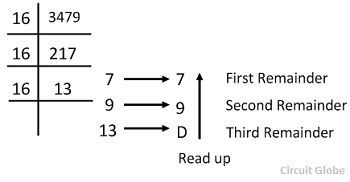The decimal number system has 10 digits from 0 – 9 and hence the base of the system is 10. On the other hand, the hexadecimal number system has 16 digits. The base of the hexadecimal number system is 16. The first 10 digits are expressed by the number and the remaining digits are represented by the alphabets. The value of the decimal number system depends on the location of the digit and the decimal points represent the fractions.

### Hexadecimal to Decimal Conversion Method

The base of the hexadecimal number system is 16, therefore the weights corresponding to various positions of the digits will be as shown below.For instance, consider the conversion of hexadecimal number E8F6.27 into its equivalent binary number.### Decimal to Hexadecimal Conversion Method

The conversion of the given decimal number into hexadecimal number requires the application of hex-dabble method which is similar to the double-dabble method with the exception that one has to divide the given number by 16 instead of 2.

Consider the conversion of the decimal number 3749 into its hexadecimal equivalent number.The third reminder 13 is equivalent to D in a hexadecimal number system. Thus the equivalent hexadecimal number D97